# On normalized distance Laplacian eigenvalues of graphs and applications to graphs defined on groups and rings

### Full PDFcarpathian_2023_39_1_213_230

The normalized distance Laplacian matrix of a connected graph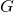, denoted by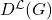, is defined by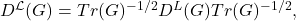where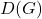is the distance matrix, the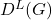is the distance Laplacian matrix and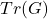is the diagonal matrix of vertex transmissions of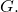The set of all eigenvalues ofincluding their multiplicities is the normalized distance Laplacian spectrum or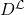-spectrum of. In this paper, we find the-spectrum of the joined union of regular graphs in terms of the adjacency spectrum and the spectrum of an auxiliary matrix. As applications, we determine the-spectrum of the graphs associated with algebraic structures. In particular, we find the-spectrum of the power graphs of groups, the-spectrum of the commuting graphs of non-abelian groups and the-spectrum of the zero-divisor graphs of commutative rings. Several open problems are given for further work.